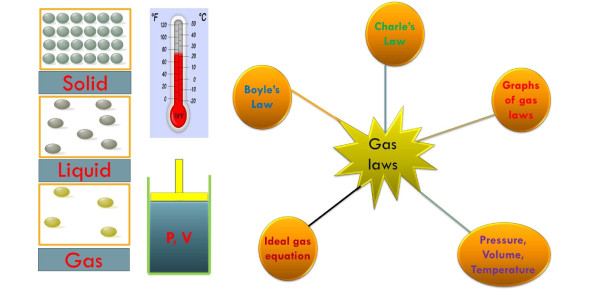# Gas Laws Quiz Questions And Answers

20 Questions | Attempts: 31174
ShareSettingsDo you know about the gas laws in chemistry? Take this gas laws quiz to see how well you know about the laws of gas. The gas laws consist of three primary laws, and they include Charles' Law, Boyle's Law, and Avogadro's Law, all of which will later combine into the General Gas Equation and Ideal Gas Law. How attentive were you when we were concerned about gas laws and their formulas in class? Take up the quiz below and get to test your understanding. All the best! Share the quiz with friends for practice

• 1.
According to Charles Law, if you have a balloon inside a car at noon during a hot summer day, the balloon molecules inside will increase in pressure.
• A.

True

• B.

False

• 2.
A nitrogen gas has occupied a volume of 500ml at a pressure of 0.971atm. What volume will the gas fill at a pressure of 1.50 atm, assuming the temperature remains constant?
• A.

342mL

• B.

424mL

• C.

324mL

• D.

442mL

• E.

242mL

• 3.
Not all Gas Law problems have Kelvin (K) as the unit of temperature. They can be expressed in Celsius (°C) and Fahrenheit(°F). So, convert 123°C to K.
• A.

396K

• B.

486K

• C.

369K

• D.

458K

• E.

693K

• 4.
Gas pressure is caused by:
• A.

Gas molecules heating up.

• B.

Gas molecules react with other gas molecules.

• C.

Gas molecules hitting the walls of a container.

• D.

Gas molecules hitting other gas molecules.

• 5.
At a pressure of 5.0 atmospheres, a sample of gas occupies 40 liters. What volume will the same sample hold at 1.0 atmosphere?
• A.

0.0050 L

• B.

0.13 L

• C.

200 L

• D.

8.0 L

• 6.
As the volume of confined gas decreases at the constant temperature, the pressure exerted by the gas ______.
• A.

Decreases

• B.

Increases

• C.

Stay the same

• D.

Fluctuates

• 7.
A sample of Argon has a volume of 0.43 mL at 299K. At what temperature in degrees Celsius will it have a volume of 1 mL.
• A.

695°C

• B.

422°C

• C.

428°C

• D.

694°C

• 8.
Each of these flasks contains the same number of molecules. In which container is the pressure highest?
• A.

• B.

• C.

• D.

• E.

• 9.
A Good example of Charles' law is when a piece of metal expands in the heat.
• A.

True

• B.

False

• 10.
Assume that the temperature remains constant. How can you increase the pressure of a gas?
• A.

Increase the container volume.

• B.

Add more molecules of the gas.

• C.

Decrease the container volume.

• D.

None of the above.

• 11.
In a closed container at 1.0 atmosphere, the temperature of a sample of gas is raised from 300 K to 400 K. What will be the final pressure of the gas?
• A.

0.010 atm

• B.

0 atm

• C.

100 atm

• D.

1.3 atm

• 12.
At constant pressure and 25°C, a sample of gas occupies 4.5 liters. At what temperature will the gas occupy 9.0 liters?
• A.

596K

• B.

50K

• C.

50°C

• D.

596°C

• 13.
You drove continuously from Laguna to Manila, and you observed that the pressure in your tires increased. This is because of the increased temperature outside the tire caused by friction.
• A.

True

• B.

False

• 14.
Each of these flasks contains the same number of gas molecules. In which would the pressure be lowest?
• A.

• B.

• C.

• D.

• 15.
Neon gas has a volume of 2,000 ml with a pressure of 1.8atm however, the pressure decreased to 1.3 atm; what is now the volume of the neon gas?
• A.

2, 795mL

• B.

2,759mL

• C.

3,795mL

• D.

3,759mL

• 16.
Each of these flasks is the same size and at the same temperature. Which one contains the most molecules?
• A.

• B.

• C.

• D.

• 17.
When a supply of hydrogen gas is held in a 4-liter container at 320 K, it exerts a pressure of 800 torrs. The supply is moved to a 2-liter container and cooled to 160 K. What is the new pressure of the confined gas?
• A.

800 torr

• B.

1600 torr

• C.

200 torr

• D.

400 torr

• 18.
Which Gas Law is involved when a balloon pops after being sat on?
• A.

Charles Law

• B.

Boyle's Law

• C.

• D.

None of the Above

• 19.
A small sample of helium gas occupies 6 mL at a temperature of 250 K. At what temperature does the volume expand to 9 mL?
• A.

125K

• B.

375K

• C.

500K

• D.

2250K

• 20.
Organize the following gasses in order of their rates of diffusion, from slowest to fastest
• oxygen, O2
• ammonia, NH3
• hydrogen, H2
• carbon dioxide, CO2
• A.

Hydrogen, oxygen, carbon dioxide, ammonia

• B.

Oxygen, hydrogen, carbon dioxide, ammonia

• C.

Hydrogen, ammonia, oxygen, carbon dioxide

• D.

Hydrogen, oxygen, ammonia, carbon dioxide

• E.

Carbon dioxide, oxygen, ammonia, hydrogen

## Related TopicsBack to top
×

Wait!
Here's an interesting quiz for you.